Courses

# 28 Year NEET Questions: Electric Charges & Fields

## 37 Questions MCQ Test Physics 28 Years Past year papers for NEET/AIPMT Class 12 | 28 Year NEET Questions: Electric Charges & Fields

Description
This mock test of 28 Year NEET Questions: Electric Charges & Fields for NEET helps you for every NEET entrance exam. This contains 37 Multiple Choice Questions for NEET 28 Year NEET Questions: Electric Charges & Fields (mcq) to study with solutions a complete question bank. The solved questions answers in this 28 Year NEET Questions: Electric Charges & Fields quiz give you a good mix of easy questions and tough questions. NEET students definitely take this 28 Year NEET Questions: Electric Charges & Fields exercise for a better result in the exam. You can find other 28 Year NEET Questions: Electric Charges & Fields extra questions, long questions & short questions for NEET on EduRev as well by searching above.
QUESTION: 1

### Point charges + 4q, –q and +4q are kept on theX-axis at points x = 0, x =a and x = 2a respectively.

Solution:

Net force on each of the charge due to the other charges is zero. However, disturbance in any direction other than along the line on which the charges lie, will not make the charges return.

QUESTION: 2

### A charge q is placed at the centre of the line joining two exactly equal positive charges Q. The system of three charges will be in equilibrium, if q is equal to

Solution:

At equilibrium, net force is zero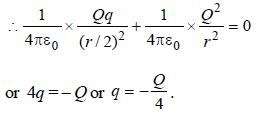QUESTION: 3

### An electric dipole, consisting of two opposite charges of 2 × 10-6 C each separated by a distance 3 cm is placed in an electric field of 2 × 105 N/C. Torque acting on the dipole is    

Solution:

Charges (q) = 2 × 10-6 C,

Distance (d) = 3 cm = 3 × 10-2 m

and electric field (E) = 2 × 105 N/C. Torque (t) = q.d.

E = (2 × 10-6) × (3 × 10-2) × (2 × 105)

= 12 × 10-3N-m.

QUESTION: 4

There is an electric field E in x-direction. If the work done on moving a charge of 0.2 C through a distance of 2 m along a line making an angle 60° with x-axis is 4J, then what is the value of E?       

Solution:

Charge (q) = 0.2 C; Distance (d) = 2m; Angle
θ = 60º and work done (W) = 4J.
Work done in moving the charge (W)
= F.d cos θ = qEd cos θ

or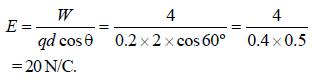QUESTION: 5

Intensity of an electric field (E) depends on distance r, due to a dipole, is related as 

Solution:

Intensity of electric field due to a Dipole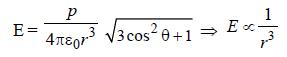QUESTION: 6

A point charge +q is placed at mid point of a cube of side ‘L’. The electric flux emerging from the cube is 

Solution:

Intensity of electric field due to a Dipole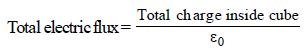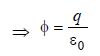QUESTION: 7

The formation of a dipole is due to two equaland dissimilar point charges placed at a

Solution:

Dipole is formed when two equal and unlike charges are placed at a short distance.

QUESTION: 8

From a point charge, there is a fixed point A. At A, there is an electric field of 500 V/m and potential difference of 3000 V. Distance between point charge and A will be 

Solution:

Given : Electric field (E) = 500 V/m
and potential difference (V) = 3000 V.
We know that electric field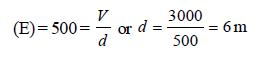[where d = Distance between point charge
and A]

QUESTION: 9

A hollow insulated conduction sphere is given a positive charge of 10 μC. What will be the electric field at the centre of the sphere if its radius is 2 metres? 

Solution:

Charge resides on the outer surface of a conducting hollow sphere of radius R. We consider a spherical surface of radius r < R.
By Gauss's theorem,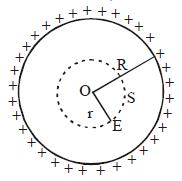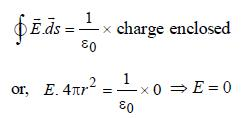i.e. electric field inside a hollow sphere is zero.

QUESTION: 10

A particle of mass m and charge q is placed atrest in a uniform electric field E and then released.The kinetic energy attained by the particle after moving a distance y is 

Solution:

K.E. = Force × distance = qE.y

QUESTION: 11

A point Q lies on the perpendicular bisector of an electrical dipole of dipole moment p. If the distance of Q from the dipole is r (much larger than the size of the dipole), then the electric field at Q is proportional to 

Solution: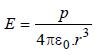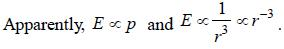QUESTION: 12

When air is replaced by a dielectric medium of force constant K, the maximum force of attraction between two charges, separated by a distance 

Solution:

in air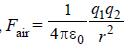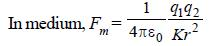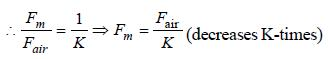QUESTION: 13

A semi-circular arc of radius ‘a’ is charged uniformly and the charge per unit length is λ. The electric field at the centre of this arc is 

Solution:

λ = linear charge density;
Charge on elementary portion dx = λ dx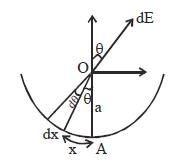Electric field at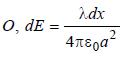Horizontal electric field, i.e., perpendicular to AO, will be cancelled.
Hence, net electric field = addition of all electrical fields in direction of AO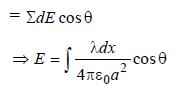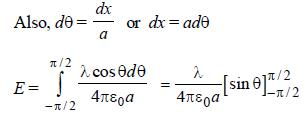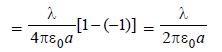QUESTION: 14

A charge Q is placed at the corner of a cube, but is completely inside the cube.The electric flux through all the six faces of the cube is 

Solution:

According to Gauss's theorem, electric flux through a closed surface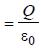where q is charge enclosed by the surface.

QUESTION: 15

The electric intensity due to a dipole of length 10 cm and having a charge of 500 μC, at a point on the axis at a distance 20 cm from one of the charges in air, is 

Solution:

Given : Length of the dipole (2l) =10cm
= 0.1m or l = 0.05 m
Charge on the dipole (q) = 500 μC = 500 × 10–6 C and distance of the point on the axis from the mid-point of the dipole (r) = 20 + 5 = 25 cm = 0.25 m. We know that the electric field intensity due to dipole on the given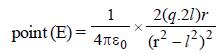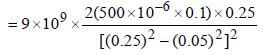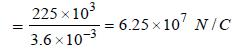QUESTION: 16

If a dipole of dipole moment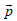is placed in a uniform electric field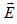, then torque acting on it is given by       

Solution:

Given : Dipole moment of the dipole =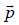and
uniform electric field =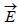We know that dipole moment (p) = q.a (where q is the charge and a is dipole length). And when a dipole of dipole momentis placed in a uniform electric field, then Torque (τ) = Either force × Perpendicular distance between the two forces = qaEsinθ or τ = pE sin θ or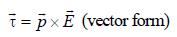QUESTION: 17

A charge QμC is placed at the centre of a cube, the flux coming out from any surface will be

Solution:

Total flux out of all six faces as per Gauss's
theorem should be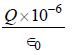Therefore, flux coming out of each face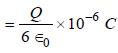QUESTION: 18

An electron is moving round the nucleus of a hydrogen atom in a circular orbit of radius r. The Coulomb force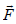between the two is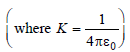Solution:

Charges (–e) on electron and (e) on proton exert a force of attraction given by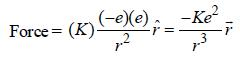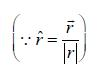Note : Magnitude of Coulomb force is given by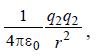but in vector form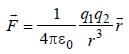QUESTION: 19

A charge q is located at the centre of a cube. The electric flux through any face is 

Solution:

Cube has 6 faces. Flux through any face is given by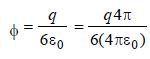QUESTION: 20

A square surface of side L metres is in the plane of the paper. A uniform electric field(volt / m), also in the plane of the paper, is limited only to the lower half of the square surface (see figure). The electric flux in SI units associated with the surface is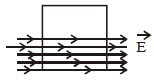Solution:

Flux =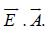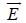is electric field vector &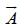is area vector

Here, angle between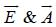is 90º.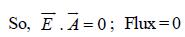QUESTION: 21

An electric dipole of moment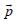is lying along a uniform electric field. The work done in rotating the dipole by 90° is

Solution:

Work done in rotating a dipole = pE (1 – cos θ)

If θ = 90º, work done = pE (1 – 0) = pE

QUESTION: 22

A hollow cylinder has a charge q coulomb within it. If φ is the electric flux in units of voltmeter associated with the curved surface B, the flux linked with the plane surface A in units of voltmeter will be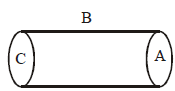Solution:

According to Gauss Law state that the net electric through the closed surface {Gaussian's surface} is equal to net charges inside the surface divided by ∈₀
Here, Let flux on A , B and C surfaces are ФA, ФB, ФC
Then, Фnet = ФA + ФB + ФC = q/∈₀
We see that , ФA = ФC and ФB = Ф
So, 2ФA + Ф = q/∈₀
ФA = 1/2(q/∈₀ - Ф)
Hence, the electric flux through plane surface is 1/2(q/∈₀ - Ф)

QUESTION: 23

Three point charges +q, –q and +q are placed at points (x = 0, y = a, z = 0), (x = 0, y = 0, z = 0) and (x = a, y = 0, z = 0) respectively. The magnitude and direction of the electric dipole moment vector of this charge assembly are 

Solution:

Three point charges +q, –2q and +q are placed at points B (x = 0, y = a, z = 0), O (x = 0, y = 0, z = 0) and A(x = a, y = 0, z = 0)
The system consists of two dipole moment vectors due to (+q and –q) and again due to (+q and –q) charges having equal magnitudes qa units – one along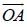and other along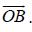Hence, net dipole moment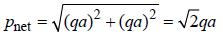along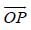at an angle 45° with positive X-axis.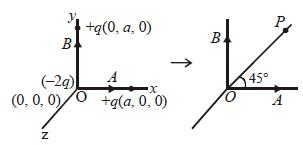QUESTION: 24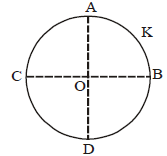A thin conducting ring of radius R is given a charge + Q. The electric field at the centre O of the ring due to the charge on the part AKB of the ring is E. The electric field at the centre due to the charge on the part ACDB of the ring is

Solution:

By the symmetry of the figure, the electric fields at O due to the portions AC and BD are equal in magnitude and opposite in direction. So, they cancel each other.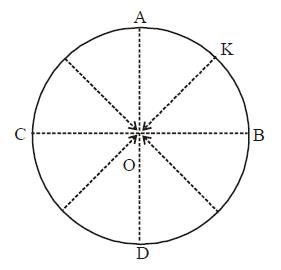Similarly, the field at O due to CD and AKB are equal in magnitude but opposite in direction. Therefore, the electric field at the centre due to the charge on the part ACDB is E along OK.

QUESTION: 25

The mean free path of electrons in a metal is 4 × 10–8 m. The electric field which can give on an average 2 eV energy to an electron in the metal will be in units of V/m   

Solution: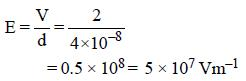QUESTION: 26

Two positive ions, each carrying a charge q, are separated by a distance d. If F is the force of repulsion between the ions, the number of electrons missing from each ion will be (e being the charge of an electron) 

Solution:

Let n be the number of electrons missing.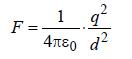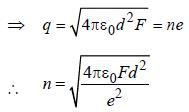QUESTION: 27

A square surface of side L meter in the plane of the paper is placed in a uniform electric field E (volt/m) acting along the same plane at an angle θ with the horizontal side of the square as shown in Figure. The electric flux linked to the surface, in units of volt. m, is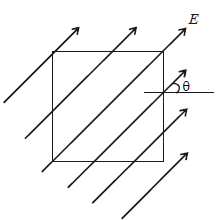Solution:

Electric flux, φ = EA cos θ , where θ = angle between E and normal to the surface.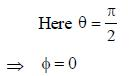QUESTION: 28

The electric field at a distance 3R/2 from the centre of a charged conducting spherical shell of radius R is E. The electric field at a distance R/2  from the centre of the sphere is

Solution:

Electric field at a point inside a charged conducting spherical shell is zero.

QUESTION: 29

A charge Q is enclosed by a Gaussian spherical surface of radius R. If the radius is doubled, then the outward electric flux will 

Solution:

By Gauss’s theorem,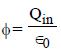Thus, the net flux depends only on the charge enclosed by the surface. Hence, there will be no effect on the net flux if the radius of the surface is doubled

QUESTION: 30

The electric potential V at any point (x, y, z), all in meters in space is given by V = 4x2 volt. The electric field at the point (1, 0, 2) in volt/meteris [2011M]

Solution: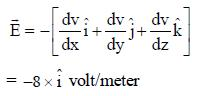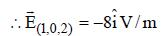QUESTION: 31

Three charges, each +q, are placed at the corners of an isosceles triangle ABC of sides BC and AC, 2a. D and E are the mid points of BC and CA. The work done in taking a charge Q from D to E is   [2011 M]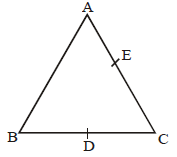Solution:

AC = BC
VD = VE
We have,
W = Q (VE – VD)

⇒W=0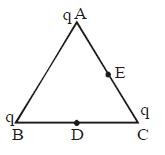QUESTION: 32

An electric dipole of moment ´p´ is placed in an electric field of intensity ´E´. The dipole acquires a position such that the axis of the dipole makes an angle θ with the direction of the field. Assuming that the potential energy of the dipole to be zero when = 90°, the torque and the potential energy of the dipole will respectively be :

Solution:

The torque on the dipole is given as τ = pE sin θ
The potential energy of the dipole in the electric field is given as
U = – pE cos θ

QUESTION: 33

What is the flux through a cube of side 'a' if a point charge of q is at one of its corner :       

Solution:

Eight identical cubes are required to arrange
so that this charge is at centre of the cube formed so flux.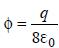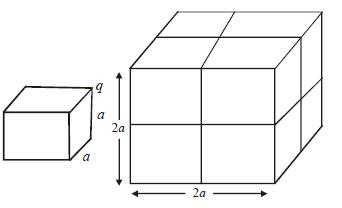QUESTION: 34

Two metallic spheres of radii 1 cm and 3 cm are given charges of –1×10–2 C and 5×10–2 C, respectively. If these are connected by a conducting wire, the final charge on the bigger sphere is : [2012M]

Solution:

At equilibrium potential of both sphere becomes same if charge of sphere one x
and other sphere Q – x then
where Q = 4 × 10–2 C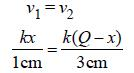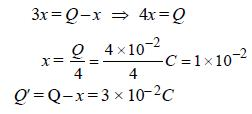QUESTION: 35

Two pith balls carrying equal charges are suspended from a common point by strings of equal length. The equilibrium separation
between them is r. Now the strings are rigidly clamped at half the height. The equilibrium separation between the balls now become
[NEET 2013]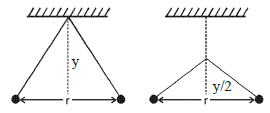Solution: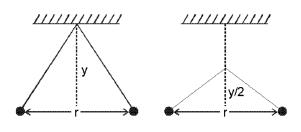From figure,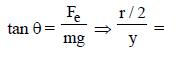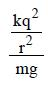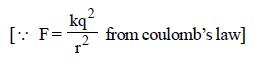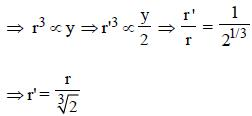QUESTION: 36

A charge ‘q’ is placed at the centre of the line joining two equal charges ‘Q’. The system of the three charges will be in equilibrium if ‘q’ is equal to

Solution:

The system of three charges will be in equilibrium.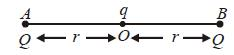For this, force between charge at A and B + force between charge at point O and either at A or B is zero.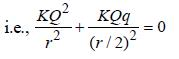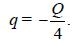QUESTION: 37

An electric dipole of dipole moment p is aligned parallel to a uniform electric field E. The energy required to rotate the dipole by 90° is   [NEET Kar. 2013]

Solution:

When electric dipole is aligned parallel θ = 0° and the dipole is rotated by 90° i.e.,
θ = 90°.
Energy required to rotate the dipole W = Uf – Ui = (–pE cos 90°) – (–pE cos 0°)
= pE.